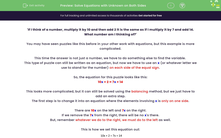# Solve Equations with Unknown on Both Sides

In this worksheet, students will learn how to solve linear equations with variables on both sides of the equals sign by grouping the terms.Key stage:  KS 4

Year:  GCSE

GCSE Subjects:   Maths

GCSE Boards:   Pearson Edexcel, OCR, Eduqas, AQA,

Curriculum topic:   Algebra

Curriculum subtopic:   Solving Equations and Inequalities Algebraic Equations

Difficulty level:#### Worksheet Overview

'If I think of a number, multiply it by 10 and then add 2 it is the same as if I multiply it by 7 and add 14. What number am I thinking of?'

You may have seen puzzles like this before in your other work with equations, but this example is more complicated.

This time the answer is not just a number, we have to do something else to find the variable.

This type of puzzle can still be written as an equation, but now we have to use an x (or whatever letter we use to stand for the number) on each side of the equal sign.

So, the equation for this puzzle looks like this:

10x + 2 = 7x + 14

This looks more complicated, but it can still be solved using the balancing method, but we just have to add an extra step.

The first step is to change it into an equation where the elements involving x is only on one side.

There are 10x on the left and 7x on the right.

If we remove the 7x from the right, there will be no x's there.

But, remember whatever we do to the right, we must do to the left as well.

This is how we set this equation out:Now, the equation should be simpler to solve.

We need to subtract 2 from both sides, then divide by 3.

Our full working will look like this:Hopefully you're following this.

Let's try another example to check.

e.g. 3x - 5 = 10 - 2x

Now this time you will notice that on the right-hand side we have 2x, which is subtracted from 10.

So, what we do in this case is add 2x to both sides first to get rid of the -2x.

Here is our full working:The rule to remember with these equations is to look for the side with the fewest x's on and take this from both sides.

Have a go at the questions and don't worry if you get them wrong, just look at the solution and remember to record all your working so you can compare.

In this activity, we will solve equations with variables on both sides of the equals sign by grouping the terms.

### What is EdPlace?

We're your National Curriculum aligned online education content provider helping each child succeed in English, maths and science from year 1 to GCSE. With an EdPlace account you’ll be able to track and measure progress, helping each child achieve their best. We build confidence and attainment by personalising each child’s learning at a level that suits them.

Get started